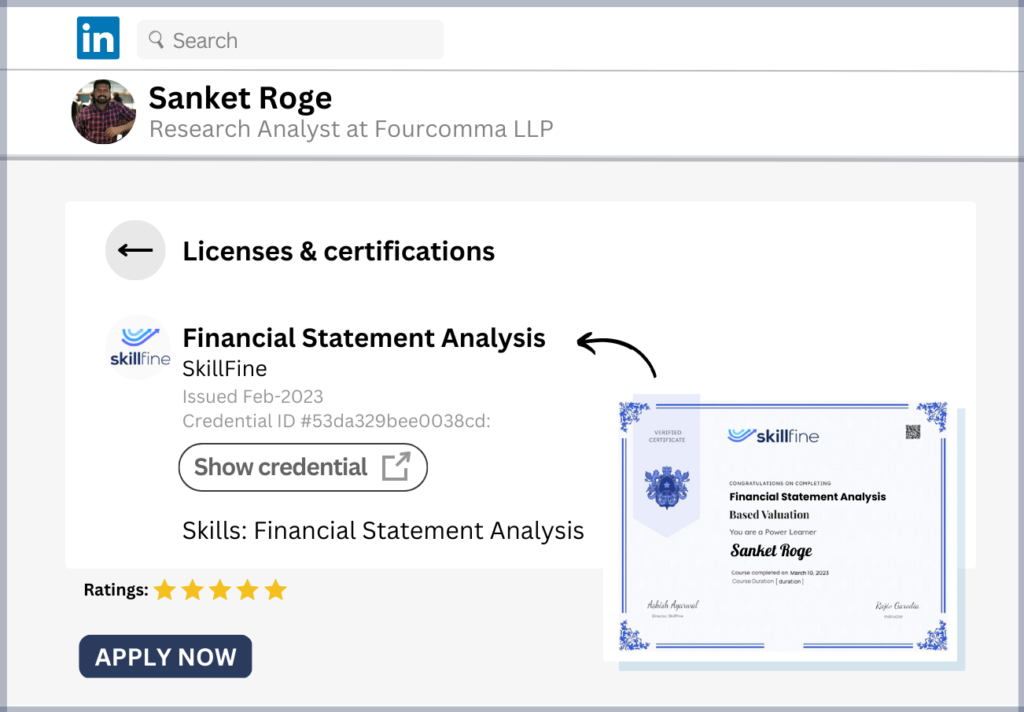# How to Do Break-Even Analysis in Excel: A Step-by-Step Guide

## How to Do Break-Even Analysis in Excel: A Step-by-Step Guide

How To Do Break Even Analysis In Excel: A Step By Step Guide

Break-even analysis is a useful tool to analyze your business and identify when you’ll be turning a profit. It will also help you manage cash flow and plan for the future. This article covers everything you need to know about break-even analysis in Excel. What is Break Even Analysis? Break Even Analysis quantifies the balance of costs and revenue required to make a project or investment profitable. It measures the point at which revenues equal to costs in order to break even. That means, there is no profit from which it can be deducted, so no loss either. Once an organization passes that balance point, it becomes profitable. Let’s look at some examples:

How to do break even in Excel?

There are a few ways to calculate the break even point in Excel. Let’s find out how. First, you need to determine the total costs of your product. To do this, you need to know all the expenses that are associated with the production of your product. This includes the purchase price of raw materials, wages of the staff, rent of the premises, electricity bill and others. You can find all this information in a proper accounting log. You can also find the break even point using a calculator. You can calculate the break even point using the following formula: Where, C = Total Cost of your product.

Calculate the fixed cost

The fixed cost is the cost that is incurred irrespective of the level of production. You can find the fixed cost in your accounting log as it may or may not be recorded as depreciation. For example, if you are producing beer, you can assume that the cost per gallon is \$2. You have to invest \$50 for the raw materials. After that, you have to hire three people for the production, and they each have to be paid \$10 per day. This means that you have to pay \$30 per day and \$120 per month for your fixed cost.

Calculate the variable cost

The variable cost is the cost that is dependent on the production level. You have to estimate all the variable costs for every single unit of your product. For example, if you are producing a beer, you will have to buy the raw materials for \$2 per gallon. Then, you will have to hire three people for the production, and they each will have to be paid \$10 per day. This means that your variable cost per unit is \$30.

Estimate the selling price for product A and B

Now, you can estimate the selling price of your product. Suppose, you want to know the break even point of selling beer at \$2 per gallon. You can assume that the total market demand is 10 gallons. Then, you have to estimate how much will be the demand of your beer after 2 weeks of its availability in the market. Now, you can calculate the market demand at the given price. Suppose, 10% of the demand is 2 gallons, then, 40% of the demand is 4 gallons, and 60% of the demand is 6 gallons. These are just examples, but you can use the same formula for all kind of products.

Sum up all the costs

Now, you need to add up all the costs that are involved in the production of your product. This includes the cost of the raw materials, the salary of the staff, electricity bill, rent, etc.

Check if you’ve reached break even point

After adding up all the costs, you need to calculate the total revenue and subtract the total costs to find the break even point. For example, if your total revenue is \$10, the cost of production is \$5. The profit per gallon is \$2 and the break even point is 10 gallons of beer. Thus, you need to sell 10 gallons of beer to make \$100 in revenue.

Draw conclusions

Now, you can draw some conclusions based on the result of your analysis. You can decide whether it is better to sell your product at a higher price or buy new machines and expand the production. You can decide whether it is better to expand your market or hire more salespeople. You can decide whether it is better to invest in advertising or invest in brand building. These are just a few examples, but you can use the same formula for all kinds of businesses.

Summary

Now, you know how to do break even analysis in Excel. To calculate the break even point, you need to determine the total costs of producing your product, add up all the variable costs for every unit, estimate the total revenue, and then subtract the cost to find the break even point. Below are some handy Excel formulas that can help you calculate the break even point of your business. Keep them in mind and you will be able to calculate the break even point of your business in no time!

1.